### Home > GC > Chapter 1 > Lesson 1.3.1 > Problem1-103

1-103.

Copy the table below, complete it, and write a rule relating $x$ and $y$. 1-103 HW eTool (Desmos).

$\left. \begin{array} { | c | c | c | c | c | c | c | } \hline x & { - 3 } & { - 2 } & { - 1 } & { 0 } & { 1 } & { 2 } & { 3 } & { 4 } \\ \hline y & { - 7 } & { } & { } & { 2 } & { 5 } & { } & { 14 } \\ \hline \end{array} \right.$

Use the $y= mx + b$ format to write your rule.

Find $m$ (the slope) by finding the difference between the $y$-values for two consecutive $x$-values.

Add the difference between the numbers (3) to the previous number in order to find each missing $y$-value.

Use this pair of numbers to solve for $b$.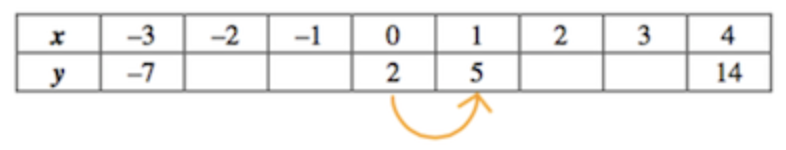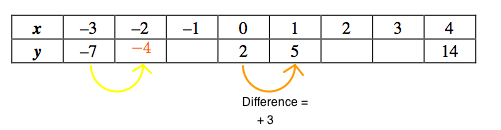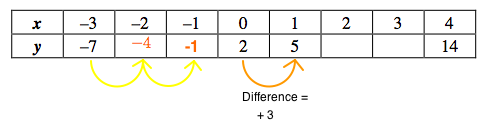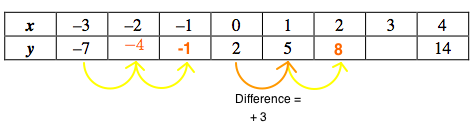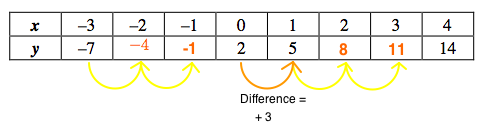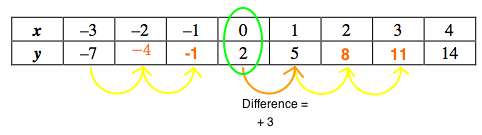$y = mx + b$

$2 = m(0) + b$

$2 = 0 + b$

$b = 2$

Substitute the values you found for $m$ and $b$ into the original equation.

$y = 3x + 2$

Use the eTool below to graph the data in the table.
Click the link at right for the full version of the eTool: GC 1-103 HW eTool